# The classic start, simple and easy in python¶

In :
print("Hello world!")

Hello world!


# Easy to use as a calculator¶

In :
2+2

Out:
4

# Python is a dynamic language, no need to declare variable types before assignment¶

In :
foo = 2   # this is a comment
bar = 2
foo+bar,foo-bar,foo*bar,foo/bar,foo**bar

Out:
(4, 0, 4, 1, 4)

# There are floats, integers, strings, lists¶

In :
a = [2.01, 3, 'hello']   # lists can contain anything,
print(a, a, a[0:2], "<--- array slicing example")   # showing list/array slicing
print(a*2, "<--- multiplying a list")               # multiplying a list
a.append('goodbye')      # adding to a list
print(a, "<--- appending a list")

([2.01, 3, 'hello'], 'hello', [2.01, 3], '<--- array slicing example')
([2.01, 3, 'hello', 2.01, 3, 'hello'], '<--- multiplying a list')
([2.01, 3, 'hello', 'goodbye'], '<--- appending a list')


# You can also import packages for useful code content, such as functions, constants, whatever¶

In :
import math   # the import function
print(math.pi)   # now you've got pi!

3.14159265359

In :
from math import *   # another way to import things, but this method isn't recommended
print(pi)

3.14159265359

In :
from numpy import pi as anotherpi  # the recommended way to import single functions
import numpy as np                 # import a package as a new name, this is the most common
print(anotherpi)
print(np.pi)

3.14159265359
3.14159265359


# Numpy is a powerful pacakge that you'll use a lot¶

In :
print(np.zeros(10))        # make an empty array
print(np.ones(10))         # make an array of ones
b = np.arange(10)          # make an array of integer indices
print(b)

[ 0.  0.  0.  0.  0.  0.  0.  0.  0.  0.]
[ 1.  1.  1.  1.  1.  1.  1.  1.  1.  1.]
[0 1 2 3 4 5 6 7 8 9]

In :
print(b*b)                  # multiplying arrays of same size
print((b*b).reshape(2,5))   # you can also reshape arrays

[ 0  1  4  9 16 25 36 49 64 81]
[[ 0  1  4  9 16]
[25 36 49 64 81]]


# numpy also has useful functions like mean, std¶

In :
testmean=10
teststd=2
ntestpoints=100
# use numpy's random number sampling from a normal distribution (which has a sigma=1)
#  to generate some fake data
testdata=np.ones(ntestpoints)*testmean + np.random.randn(ntestpoints)*teststd
themean = np.sum(testdata)/ntestpoints   # calculating the mean without numpy
stderr = np.sqrt( 1./(ntestpoints-1) * np.sum( (np.mean(testdata)-testdata)**2) )   #calculating the std error
error_on_mean = stderr/(sqrt(ntestpoints))
print "The mean: "+ str(np.mean(testdata))+", or "+str(themean)    # our mean and numpy's mean should be the same
print "The standard error: " +str(np.std(testdata,ddof=1))+", or "+str(stderr)  #note the ddof=1
print "The error on the mean: " + str(error_on_mean)

The mean: 9.86443753209, or 9.86443753209
The standard error: 1.88782447342, or 1.88782447342
The error on the mean: 0.188782447342


# Plotting is inside the matplotlib.pyplot package¶

In :
import matplotlib.pyplot as plt
%pylab inline

Populating the interactive namespace from numpy and matplotlib

/home/andrew/.local/lib/python2.7/site-packages/IPython/core/magics/pylab.py:161: UserWarning: pylab import has clobbered these variables: ['cosh', 'ldexp', 'hypot', 'tan', 'isnan', 'log', 'fabs', 'floor', 'modf', 'sqrt', 'frexp', 'degrees', 'pi', 'log10', 'sin', 'fmod', 'copysign', 'cos', 'ceil', 'isinf', 'sinh', 'bar', 'trunc', 'expm1', 'e', 'tanh', 'radians', 'exp', 'log1p', 'gamma']
%matplotlib prevents importing * from pylab and numpy
"\n%matplotlib prevents importing * from pylab and numpy"

In :
plt.figure(figsize=(10,6))
plt.hist(testdata,bins=ntestpoints/10,histtype='stepfilled',alpha=.5,color='g',
range=[5,15])
plt.xlabel('Value',fontsize=20)
plt.ylabel('Number of measurements \n in each bin',fontsize=20)
plt.title('Histogram of test data',fontsize=22)
plt.axvline(themean,linestyle='-',color='r')
plt.axvline(themean+error_on_mean,linestyle='--',color='b')
plt.axvline(themean-error_on_mean,linestyle='--',color='b')
plt.axvline(testmean,color='k',linestyle='-')

Out:
<matplotlib.lines.Line2D at 0x7fe8bedab0d0>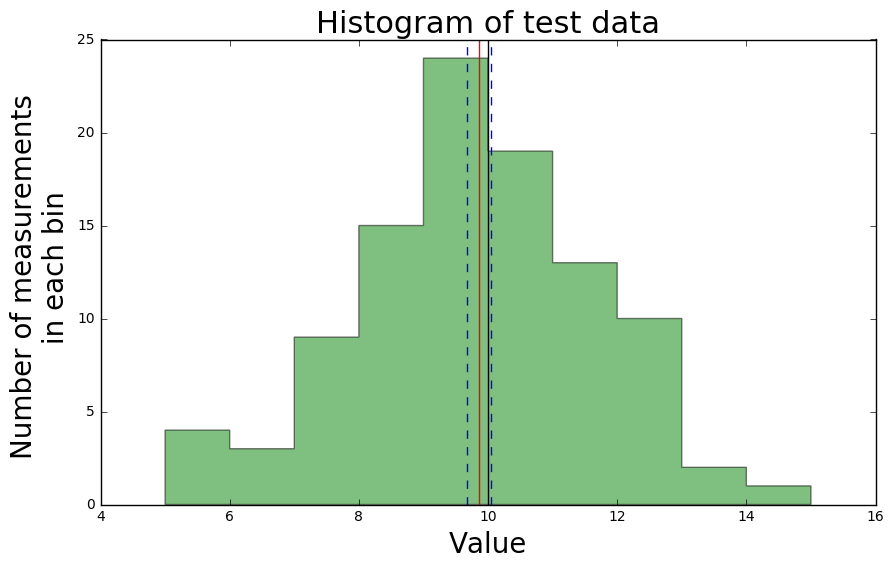# You can also read in data from a text file with numpy's loadtxt function¶

In :
# Click on the menu for the graph on
# and put its filename below

# this data has four columns
#  date, cowboys searches, patriots searches, superbowl searches

# Use numpy's recfromcsv function to read in the csv file from Google
# another option is to use np.loadtxt
# (an example of that syntax is at the end of this notebook)
# the skip_header call is to skip a few lines at the top that aren't data
# and the names allow us to now reference the records using a variable name rather than integers

timedat=np.array([np.array(int(datedat.split('-'))+int(datedat.split('-'))/12.) for datedat in goog['week']])
#this translates the "2004-01" string into fractional years as a floating point number

plt.figure(figsize=(10,3))
for datname in ['cowboys','pats','supabowl']:
plt.plot(timedat,goog[datname],label=datname,linewidth=2,alpha=.7)
plt.legend(loc='upper center',fontsize=10)
#plt.yscale('log')
plt.ylabel('Google interest [arb. units]',fontsize=12)
plt.xlabel('Time since 0 BCE [years]',fontsize=12)
plt.title('Hand-egg sports teams and their championships \n as measured through Google search interest')

plt.xticks(np.arange(2004,2018))
print ('how bout dem cowboys')

# put a vertical line for every patriots superbowl visit
patsup=[2004,2007,2011,2014] #years they went to the superbowl
for goodyear in patsup:  # loop over them
plt.axvline(goodyear+1.2,color='k')

how bout dem cowboys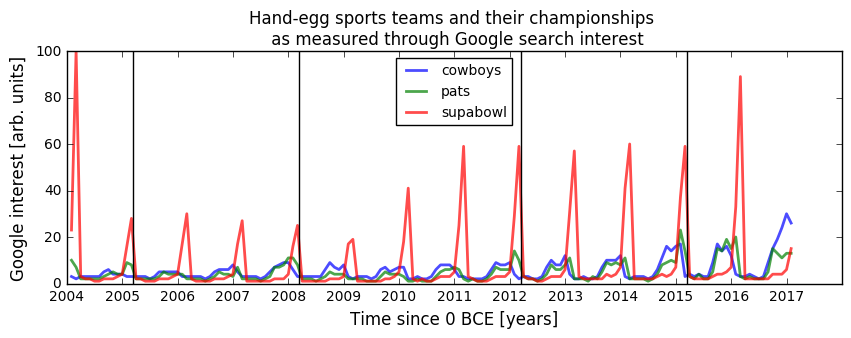# You can define functions to operate on variables¶

In :
# start function definitions with the "def " and the name of the function
# inside the parentheses you place the variables which squared takes in
def squared(thingtosquare):
"""This is a documentation string for the function squared, which takes
in a number (thingtosquare) and outputs its square (thingsquared)"""
thingsquared = thingtosquare ** 2     # simply squares the input variable, and returning it
return thingsquared

In :
squared(4)

Out:
16
In :
print squared(b),help(squared)

[ 0  1  4  9 16 25 36 49 64 81]Help on function squared in module __main__:

squared(thingtosquare)
This is a documentation string for the function squared, which takes
in a number (thingtosquare) and outputs its square (thingsquared)

None

In :
# define a function to make a gaussian with input values, used later
def mygauss(x, amp, center, sigma):
"""This is an example gaussian function, which takes in x values, the amplitude (amp),
the center x value (center) and the sigma of the Gaussian, and returns the respective y values."""
y = amp * np.exp(-.5*((x-center)/sigma)**2)
return y

In :
npts = 40   # the number of points on the x axis
x = np.linspace(0,10,npts)    # make a series of npts linearly spaced values between 0 and 10
amp = 3.1
center = 5.1
sigma = 1.11
y = mygauss(x, amp, center, sigma)
print y

[  8.07827074e-05   2.27337292e-04   6.06524751e-04   1.53409389e-03
3.67858601e-03   8.36248704e-03   1.80225221e-02   3.68231757e-02
7.13267572e-02   1.30981297e-01   2.28029826e-01   3.76356649e-01
5.88888531e-01   8.73558844e-01   1.22850464e+00   1.63789856e+00
2.07024987e+00   2.48075627e+00   2.81819471e+00   3.03517306e+00
3.09899937e+00   2.99975015e+00   2.75279737e+00   2.39490875e+00
1.97528272e+00   1.54452564e+00   1.14495007e+00   8.04643554e-01
5.36100352e-01   3.38621254e-01   2.02771961e-01   1.15113737e-01
6.19543740e-02   3.16113001e-02   1.52910852e-02   7.01228930e-03
3.04864536e-03   1.25654936e-03   4.90995791e-04   1.81886953e-04]

In :
plt.figure(figsize=(10,6))
plt.plot(x,y,'bo', label='data points')
plt.text(center, amp, "<-- peak is here",fontsize=16)     # places text at any x/y location on the graph
plt.xlabel('X axis',fontsize=20)
plt.ylabel('Y axis', fontsize=20)
plt.title('A gaussian plot \n with some extras!',fontsize=20)
plt.legend(loc='best')

Out:
<matplotlib.legend.Legend at 0x7fe8c1144790>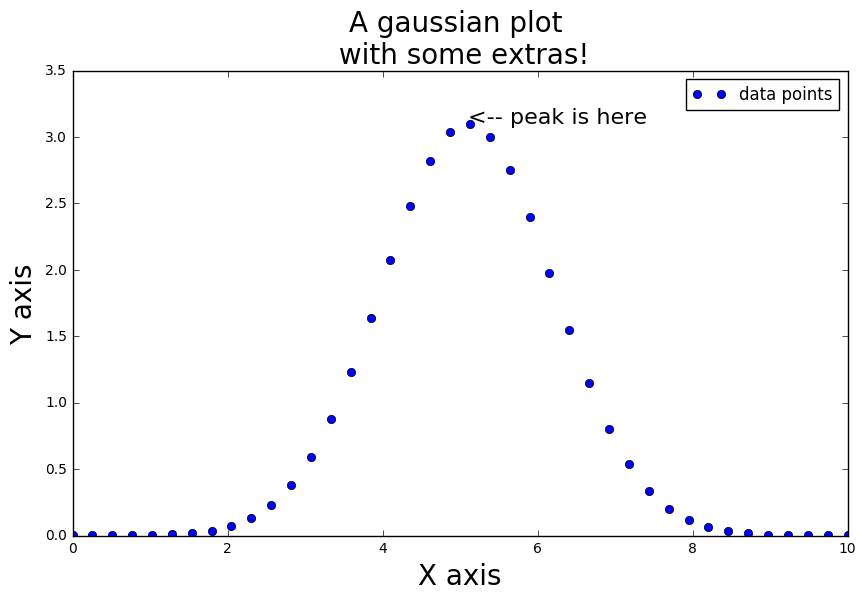# Now let's fake some measurement process by adding some errors, and fit this with a model¶

### Simulate some data, using a Gaussian with random noise added¶

In :
def mygauss_errors(x, amp, center, sigma, noise_amp):
"""Takes in x values, amplitude,center,sigma for a guassian, as well as a noise amplitude
which multiplies a random normally distributed (sigma=1,center=0) noise that is added to the gaussian"""
y = amp * np.exp(-.5*((x-center)/sigma)**2) #+ np.random.randn(len(x))
yerr = y + np.random.randn(len(x)) * noise_amp
return yerr

# make up some hidden parameters for the noisy Gaussian, we will try to discover them with optimization later
npts, minx, maxx = 15,0,10
x = linspace(minx,maxx,npts)    # making linearly spaced x values between min and max with n points
amp, center, sigma = 50.1,5.3,1.11   # made up hidden parameters
noise_amp = .05*amp   # this multiplies a unit normal distribution to simulate noise, larger means increased noise

# get the noisy y values using the above input parameters
yerr = mygauss_errors(x, amp, center, sigma, noise_amp)

## for plotting purposeses, also make a more "continuous" x variable, with much finer spacing
xcont=linspace(0,10,1000)
ycont = mygauss(xcont,amp,center,sigma) # also get the true continuous y values

plt.figure(figsize=(8,4))
plt.plot(xcont,ycont,'r',label='True values (nature)')

plt.errorbar(x,yerr,yerr=noise_amp,marker='o',linestyle='None',label='Noisy data')
plt.title('Fake data example with a noisy Gaussian')
plt.legend()

Out:
<matplotlib.legend.Legend at 0x7fe850e63d90>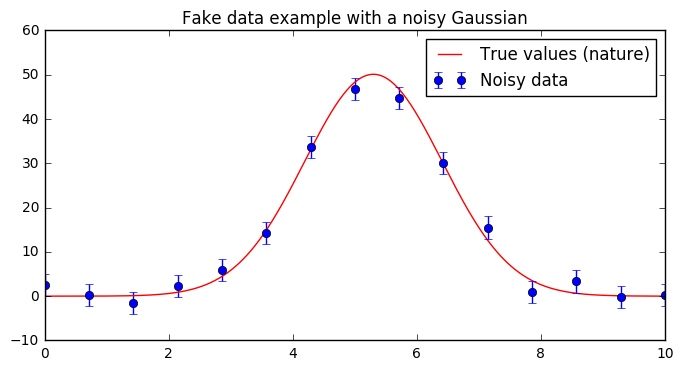# Now that we have some fake data, let's pretend we don't know the parameters and try to find them via $\chi^2$ minimization!¶

In :
# to fit a function to some data, you will need to minimize (optimize) some statistic
# scipy has a function in it which will take in a model and data and find the best parameters
# for you using a chi-squared minimization
from scipy.optimize import curve_fit

# you can always google for more info about common functions, but python has documentation strings
# that you can access in the notebook by typing

help(curve_fit)

# or also executing "curve_fit?" would pop up a window with the same information

Help on function curve_fit in module scipy.optimize.minpack:

curve_fit(f, xdata, ydata, p0=None, sigma=None, absolute_sigma=False, check_finite=True, bounds=(-inf, inf), method=None, jac=None, **kwargs)
Use non-linear least squares to fit a function, f, to data.

Assumes ydata = f(xdata, *params) + eps

Parameters
----------
f : callable
The model function, f(x, ...).  It must take the independent
variable as the first argument and the parameters to fit as
separate remaining arguments.
xdata : An M-length sequence or an (k,M)-shaped array
for functions with k predictors.
The independent variable where the data is measured.
ydata : M-length sequence
The dependent data --- nominally f(xdata, ...)
p0 : None, scalar, or N-length sequence, optional
Initial guess for the parameters.  If None, then the initial
values will all be 1 (if the number of parameters for the function
can be determined using introspection, otherwise a ValueError
is raised).
sigma : None or M-length sequence, optional
If not None, the uncertainties in the ydata array. These are used as
weights in the least-squares problem
i.e. minimising np.sum( ((f(xdata, *popt) - ydata) / sigma)**2 )
If None, the uncertainties are assumed to be 1.
absolute_sigma : bool, optional
If False, sigma denotes relative weights of the data points.
The returned covariance matrix pcov is based on *estimated*
errors in the data, and is not affected by the overall
magnitude of the values in sigma. Only the relative
magnitudes of the sigma values matter.

If True, sigma describes one standard deviation errors of
the input data points. The estimated covariance in pcov is
based on these values.
check_finite : bool, optional
If True, check that the input arrays do not contain nans of infs,
and raise a ValueError if they do. Setting this parameter to
False may silently produce nonsensical results if the input arrays
do contain nans. Default is True.
bounds : 2-tuple of array_like, optional
Lower and upper bounds on independent variables. Defaults to no bounds.
Each element of the tuple must be either an array with the length equal
to the number of parameters, or a scalar (in which case the bound is
taken to be the same for all parameters.) Use np.inf with an
appropriate sign to disable bounds on all or some parameters.

method : {'lm', 'trf', 'dogbox'}, optional
Method to use for optimization.  See least_squares for more details.
Default is 'lm' for unconstrained problems and 'trf' if bounds are
provided. The method 'lm' won't work when the number of observations
is less than the number of variables, use 'trf' or 'dogbox' in this
case.

jac : callable, string or None, optional
Function with signature jac(x, ...) which computes the Jacobian
matrix of the model function with respect to parameters as a dense
array_like structure. It will be scaled according to provided sigma.
If None (default), the Jacobian will be estimated numerically.
String keywords for 'trf' and 'dogbox' methods can be used to select
a finite difference scheme, see least_squares.

kwargs
Keyword arguments passed to leastsq for method='lm' or
least_squares otherwise.

Returns
-------
popt : array
Optimal values for the parameters so that the sum of the squared error
of f(xdata, *popt) - ydata is minimized
pcov : 2d array
The estimated covariance of popt. The diagonals provide the variance
of the parameter estimate. To compute one standard deviation errors
on the parameters use perr = np.sqrt(np.diag(pcov)).

How the sigma parameter affects the estimated covariance
depends on absolute_sigma argument, as described above.

If the Jacobian matrix at the solution doesn't have a full rank, then
'lm' method returns a matrix filled with np.inf, on the other hand
'trf'  and 'dogbox' methods use Moore-Penrose pseudoinverse to compute
the covariance matrix.

Raises
------
ValueError
if either ydata or xdata contain NaNs, or if incompatible options
are used.

RuntimeError
if the least-squares minimization fails.

OptimizeWarning
if covariance of the parameters can not be estimated.

--------
least_squares : Minimize the sum of squares of nonlinear functions.
stats.linregress : Calculate a linear least squares regression for two sets
of measurements.

Notes
-----
With method='lm', the algorithm uses the Levenberg-Marquardt algorithm
through leastsq. Note that this algorithm can only deal with
unconstrained problems.

Box constraints can be handled by methods 'trf' and 'dogbox'. Refer to
the docstring of least_squares for more information.

Examples
--------
>>> import numpy as np
>>> from scipy.optimize import curve_fit
>>> def func(x, a, b, c):
...     return a * np.exp(-b * x) + c

>>> xdata = np.linspace(0, 4, 50)
>>> y = func(xdata, 2.5, 1.3, 0.5)
>>> ydata = y + 0.2 * np.random.normal(size=len(xdata))

>>> popt, pcov = curve_fit(func, xdata, ydata)

Constrain the optimization to the region of 0 < a < 3, 0 < b < 2
and 0 < c < 1:

>>> popt, pcov = curve_fit(func, xdata, ydata, bounds=(0, [3., 2., 1.]))


In :
# use the curve_fit function to find optimal parameters for the mygauss function, given the data
# (it does better with a good guess for starting parameters, though none or even bad guesses work)
ampguess,centerguess,sigmaguess=42,4.2,.42
params,cov=curve_fit(mygauss,x,yerr,p0=[ampguess,centerguess,sigmaguess])

print("These are the best fit amplitude, center, and sigma")
print(params)
print("These are the covariances between those parameters (i.e. diagonal elements are sigma**2 for each param.)")
print(cov)

# these will print out the values and their errors, according to the covariance matrix
print "True amplitude, center, and sigma: " +str(amp)+", "+str(center)+', '+str(sigma)
print "Fitted amplitude, center, and sigma: " +str(params)
print "With estimated errors +/- " +str(np.diag(cov))
chi2 = 1./(len(x)-3)*np.sum((yerr-mygauss(x,*params))**2/(noise_amp)**2)
print 'Reduced chi-squared of fit: '+str(chi2)

These are the best fit amplitude, center, and sigma
[ 48.25156428   5.30305646   1.1577862 ]
These are the covariances between those parameters (i.e. diagonal elements are sigma**2 for each param.)
[[  1.51140205e+00   1.14560249e-09  -2.41771648e-02]
[  1.14560249e-09   1.16025091e-03  -1.44802759e-11]
[ -2.41771648e-02  -1.44802759e-11   1.16025105e-03]]
True amplitude, center, and sigma: 50.1, 5.3, 1.11
Fitted amplitude, center, and sigma: [ 48.25156428   5.30305646   1.1577862 ]
With estimated errors +/- [  1.51140205e+00   1.16025091e-03   1.16025105e-03]
Reduced chi-squared of fit: 0.461322203984

In :
str(zip(params,np.diag(cov)))

Out:
'[(48.251564279071438, 1.5114020525699201), (5.3030564570108174, 0.001160250911266647), (1.1577861962228788, 0.0011602510452089643)]'

# Now plot all the curves together: the noisy data, the true nature, and the fit¶

In :
plt.figure(figsize=(10,6))

plt.errorbar(x,yerr,yerr=noise_amp,marker='o',linestyle='None',label='Noisy data')

plt.plot(xcont,mygauss(xcont,amp,center,sigma),label='True data (nature)')
fiterrs=np.sqrt(np.diag(cov))
plt.fill_between(xcont,mygauss(xcont,*params-fiterrs),mygauss(xcont,*params+fiterrs),color='orange',alpha=.5)
plt.plot(xcont,mygauss(xcont,*params),label='Fitted curve',color='r')
plt.axhline(0, color='k', linestyle='--')
plt.xlabel('X axis',fontsize=20)
plt.ylabel('Y axis', fontsize=20)
plt.title('Our result: A noisy Gaussian with fit',fontsize=20)
plt.legend(loc='upper left')

Out:
<matplotlib.legend.Legend at 0x7fe850d22fd0>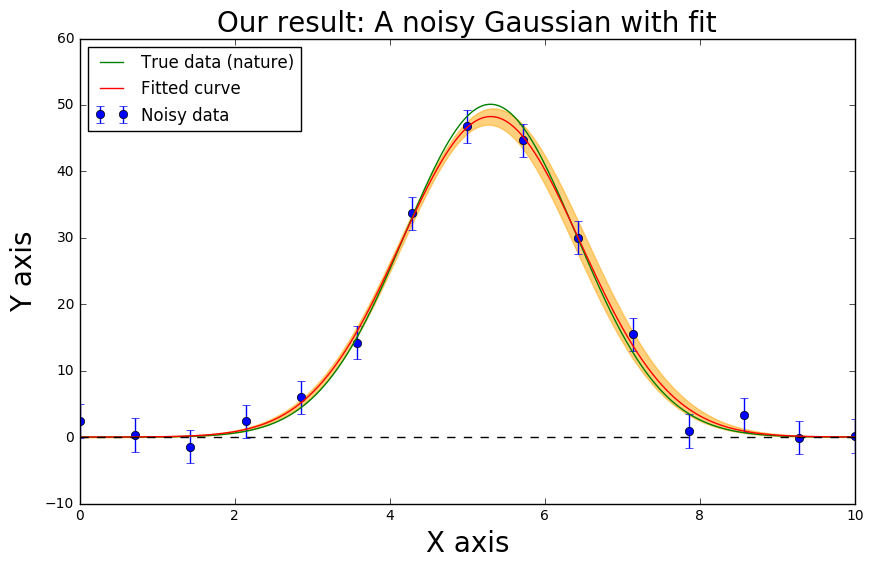### time is important, some time is worth keeping track¶

In :
import time
print time.time()   #one way to keep track of time is to use the system time
time.time?

1484158377.16


## Just for fun... we can repeat all the above, faking the data, and fitting it as many times as we want. Does it return reasonable model fits? This is a way of checking the measurement/modeling process for bias. Hint: lower/raise the number of points (npts) and noise amplitude (noise_amp) variables below to see how e.g. the reduced chi-squared distribution changes¶

In :
npts, minx, maxx = 100,0,9
x = linspace(minx,maxx,npts)    # making linearly spaced x values between min and max with n points
amp, center, sigma = 50.1,5.3,1.11   # made up hidden parameters
noise_amp = 2   # this multiplies a unit normal distribution to simulate noise, larger means increased noise

# Now just repeat the experiment with all the above true parameters
# and store the resulting model parameters
nexpts=10000    # the number of times to run the expt, 10k takes a few seconds
allchi2=np.zeros(nexpts)
allamp,allcen,allsig=np.zeros(nexpts),np.zeros(nexpts),np.zeros(nexpts)
allampstd,allcenstd,allsigstd=np.zeros(nexpts),np.zeros(nexpts),np.zeros(nexpts)

tstart=time.time()   #mark the start time
for i in range(nexpts):  # simply loop over repeated "experiments", saving the model parameters each time
yerr = mygauss_errors(x, amp, center, sigma, noise_amp)   # fake the data
params,cov=curve_fit(mygauss,x,yerr,p0=[ampguess,centerguess,sigmaguess])    # fit the model
allchi2[i]=1./(len(x)-3)*np.sum((yerr-mygauss(x,*params))**2/(noise_amp)**2)  # record chi2
allamp[i],allcen[i],allsig[i]=params    # save parameters
allampstd[i],allcenstd[i],allsigstd[i]=np.array([np.sqrt(cov[diag][diag]) for diag in range(3)])

print "took "+str(time.time()-tstart)+" seconds"   #current time minus start time is total time to run

took 4.98560094833 seconds


### there are lots of things we could now plot... for instance, is the reduced chi2 distribution reasonable?¶

In :
plt.hist(allchi2,bins=100,histtype='step')
plt.xlabel('$\\chi^2_r$',fontsize=20)
plt.title('The $\\chi^2$ of '+str(nexpts)+' repeated "measurement simulations"')
plt.axvline(1,color='k')

Out:
<matplotlib.lines.Line2D at 0x7fe85094e310>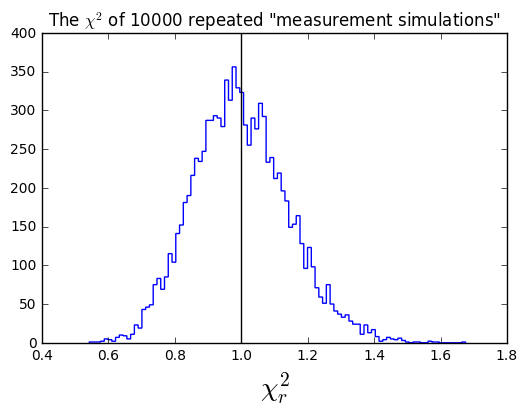### looking at the distribution of model fits and residuals¶

In :
nplots=50
thetruey=mygauss(xcont,amp,center,sigma)
plt.figure(figsize=(10,4))
for i in range(nplots):
plt.subplot(121)

thefity=mygauss(xcont,allamp[i],allcen[i],allsig[i])
plt.plot(xcont,thefity,'b.',alpha=.01)                     #plot the best fit model for this expt
plt.subplot(122)
plt.plot(xcont,(thefity-thetruey)/amp*100.,'k.',alpha=.01)  #plot the residuals

plt.ylabel('% difference')
plt.title('residuals of best fit model minus truth')
plt.axhline(0,color='k',linestyle='-',label='residuals')
plt.xlabel('x')
plt.legend()
plt.subplot(121)
plt.plot(xcont,ycont,'k',linewidth=2,label='true nature') #plot the true nature being measured
plt.title('spread of best fit model \n in repeated experiments')
plt.legend()

Out:
<matplotlib.legend.Legend at 0x7fe85098a250>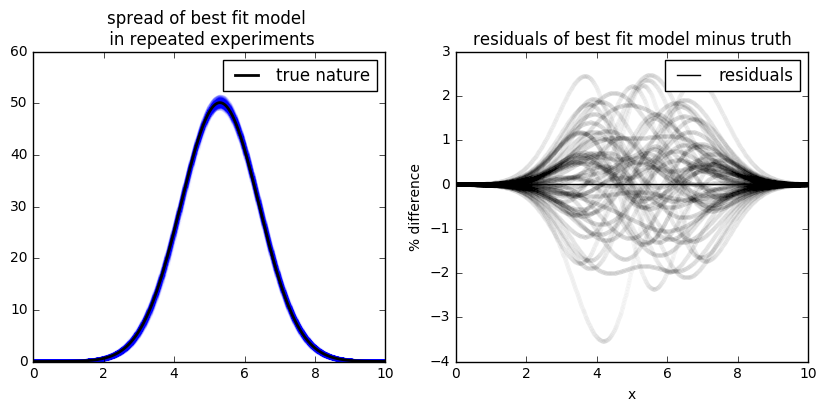In :
plt.figure(figsize=(12,3))
plt.suptitle('Reported parameter fitting "errors"')
plt.subplot(131)
plt.hist(allampstd)
xlabel('amplitude')
plt.subplot(132)
plt.hist(allcenstd)
plt.xlabel('center')
plt.subplot(133)
plt.hist(allsigstd)
plt.xlabel('sigma')
# why is the std for sigma and center the same??

Out:
<matplotlib.text.Text at 0x7fe8500a1cd0>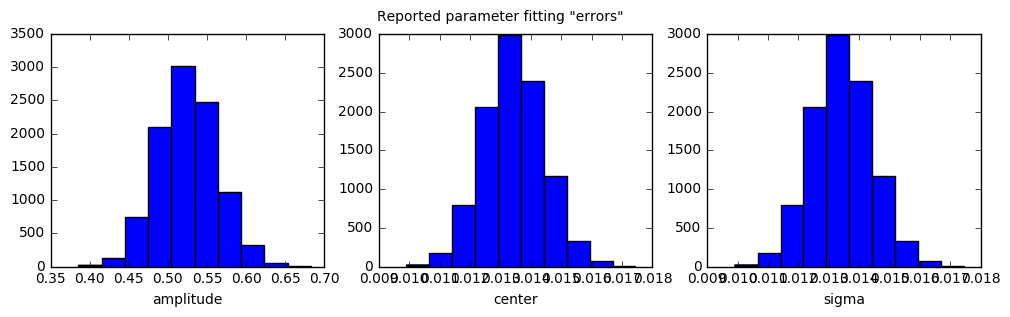In :
#This was plotting up Xiangdong's interesting lab data for the first class

xdat = np.recfromtxt('/home/andrew/Work/Data sorted by Voltage.txt',skip_header=2,
names=['n','pvol','mvol','foo','bar'])

plt.figure(figsize=(10,4))
plt.subplot(121)
plt.plot(xdat['n'],xdat['pvol'],label='+10 V',alpha=.7)
plt.plot(xdat['n'],xdat['mvol'],label='-10 V',alpha=.7)
plt.xlabel('Measurement #')
plt.ylabel('Voltage [$10^-3 V$]')
plt.legend()
plt.subplot(122)
plt.hist(xdat['pvol'],bins=100,histtype='step',label='+10 V')
plt.hist(xdat['mvol'],bins=100,histtype='step',label='-10 V')
pvmed,nvmed=np.median(xdat['pvol']),np.median(xdat['mvol'])
pvstd,nvstd=np.std(xdat['pvol'])/np.sqrt(len(xdat)),np.std(xdat['mvol'])/np.sqrt(len(xdat))
print("The median of +10/-10 voltage measurements is:",pvmed,nvmed)
print("The standard error is:",pvstd,nvstd)
plt.axvline(0,color='k')
plt.axvline(pvmed,color='b')
plt.axvline(nvmed,color='g')
plt.xlabel('millivolts')
plt.legend()
plt.suptitle("WELCOME TO $\\rho\\hbar\\psi s 122$",fontsize=30)

('The median of +10/-10 voltage measurements is:', -6.9600000000000003e-06, 4.9099999999999996e-06)
('The standard error is:', 2.6421533574604636e-06, 2.7054565169812322e-06)

Out:
<matplotlib.text.Text at 0x7fe85196df50>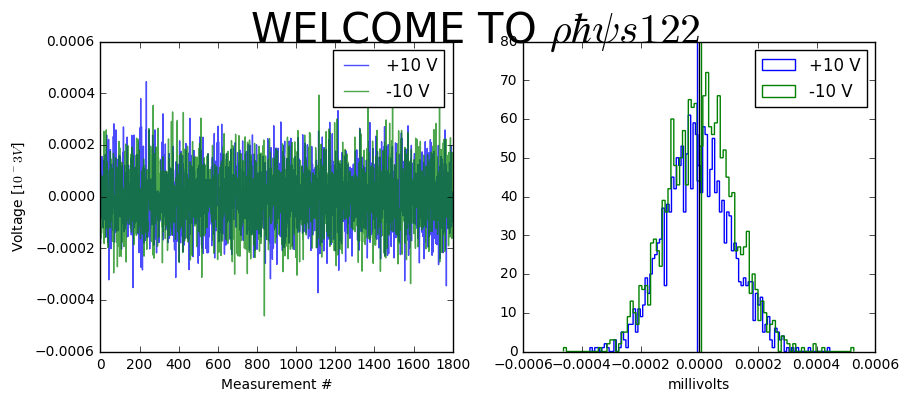In [ ]: